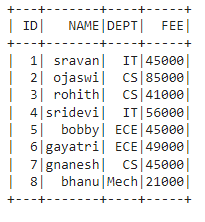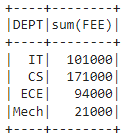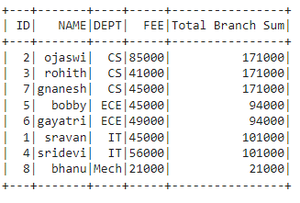# Pyspark dataframe: Summing column while grouping over another

• Last Updated : 29 Dec, 2021

In this article, we will discuss how to sum a column while grouping another in Pyspark dataframe using Python.

## Python3

 `# importing module` `import` `pyspark`   `# importing sparksession from pyspark.sql module` `from` `pyspark.sql ``import` `SparkSession`   `# creating sparksession and giving an app name` `spark ``=` `SparkSession.builder.appName(``'sparkdf'``).getOrCreate()`   `# list  of student  data` `data ``=` `[[``"1"``, ``"sravan"``, ``"IT"``, ``45000``],` `        ``[``"2"``, ``"ojaswi"``, ``"CS"``, ``85000``],` `        ``[``"3"``, ``"rohith"``, ``"CS"``, ``41000``],` `        ``[``"4"``, ``"sridevi"``, ``"IT"``, ``56000``],` `        ``[``"5"``, ``"bobby"``, ``"ECE"``, ``45000``],` `        ``[``"6"``, ``"gayatri"``, ``"ECE"``, ``49000``],` `        ``[``"7"``, ``"gnanesh"``, ``"CS"``, ``45000``],` `        ``[``"8"``, ``"bhanu"``, ``"Mech"``, ``21000``]` `        ``]`   `# specify column names` `columns ``=` `[``'ID'``, ``'NAME'``, ``'DEPT'``, ``'FEE'``]`   `# creating a dataframe from the lists of data` `dataframe ``=` `spark.createDataFrame(data, columns)`   `# display` `dataframe.show()`

Output:## Method 1: Using groupBy() Method

In PySpark,  groupBy() is used to collect the identical data into groups on the PySpark DataFrame and perform aggregate functions on the grouped data. Here the aggregate function is sum().

sum(): This will return the total values for each group.

Syntax: dataframe.groupBy(‘column_name_group’).sum(‘column_name’)

## Python3

 `# importing module` `import` `pyspark`   `# importing sparksession from pyspark.sql module` `from` `pyspark.sql ``import` `SparkSession`   `# creating sparksession and giving an app name` `spark ``=` `SparkSession.builder.appName(``'sparkdf'``).getOrCreate()`   `# list  of student  data` `data ``=` `[[``"1"``, ``"sravan"``, ``"IT"``, ``45000``],` `        ``[``"2"``, ``"ojaswi"``, ``"CS"``, ``85000``],` `        ``[``"3"``, ``"rohith"``, ``"CS"``, ``41000``],` `        ``[``"4"``, ``"sridevi"``, ``"IT"``, ``56000``],` `        ``[``"5"``, ``"bobby"``, ``"ECE"``, ``45000``],` `        ``[``"6"``, ``"gayatri"``, ``"ECE"``, ``49000``],` `        ``[``"7"``, ``"gnanesh"``, ``"CS"``, ``45000``],` `        ``[``"8"``, ``"bhanu"``, ``"Mech"``, ``21000``]` `        ``]`   `# specify column names` `columns ``=` `[``'ID'``, ``'NAME'``, ``'DEPT'``, ``'FEE'``]`   `# creating a dataframe from the lists of data` `dataframe ``=` `spark.createDataFrame(data, columns)`   `# Groupby with DEPT along FEE with sum()` `dataframe.groupBy(``'DEPT'``).``sum``(``'FEE'``).show()`

Output:## Method 2: Using agg() function with GroupBy()

Here we have to import the sum function from sql.functions  module to be used with the aggregate method.

Syntax: dataframe.groupBy(“group_column”).agg(sum(“column_name”))

where,

• dataframe is the pyspark dataframe
• group_column is the grouping column
• column_name is the column to get sum

## Python3

 `# importing module` `import` `pyspark`   `# importing sparksession from pyspark.sql module` `from` `pyspark.sql ``import` `SparkSession`   `# import sum` `from` `pyspark.sql.functions ``import` `sum`   `# creating sparksession and giving an app name` `spark ``=` `SparkSession.builder.appName(``'sparkdf'``).getOrCreate()`   `# list  of student  data` `data ``=` `[[``"1"``, ``"sravan"``, ``"IT"``, ``45000``],` `        ``[``"2"``, ``"ojaswi"``, ``"CS"``, ``85000``],` `        ``[``"3"``, ``"rohith"``, ``"CS"``, ``41000``],` `        ``[``"4"``, ``"sridevi"``, ``"IT"``, ``56000``],` `        ``[``"5"``, ``"bobby"``, ``"ECE"``, ``45000``],` `        ``[``"6"``, ``"gayatri"``, ``"ECE"``, ``49000``],` `        ``[``"7"``, ``"gnanesh"``, ``"CS"``, ``45000``],` `        ``[``"8"``, ``"bhanu"``, ``"Mech"``, ``21000``]` `        ``]`   `# specify column names` `columns ``=` `[``'ID'``, ``'NAME'``, ``'DEPT'``, ``'FEE'``]`   `# creating a dataframe from the lists of data` `dataframe ``=` `spark.createDataFrame(data, columns)`   `# Groupby with DEPT and NAME with sum()` `dataframe.groupBy(``"DEPT"``).agg(``sum``(``"FEE"``)).show()`

Output:## Method 3: Using Window function with sum

The window function is used for partitioning the columns in the dataframe.

Syntax: Window.partitionBy(‘column_name_group’)

where, column_name_group is the column that contains multiple values for partition

We can partition the data column that contains group values and then use the aggregate function of sum() to get the sum of the grouping(partitioning) column.

Syntax: dataframe.withColumn(‘New_Column_name’, functions.sum(‘column_name’).over(Window.partitionBy(‘column_name_group’)))

where,

• withColumn() method is used to get the column name
• functions.sum(‘column_name’) is to get the sum
• Window.partitionBy(‘column_name_group’) is to partition the column with sum by group

Example: Get the sum of fee based on the department

## Python3

 `# importing module` `import` `pyspark`   `# importing sparksession from pyspark.sql module` `from` `pyspark.sql ``import` `SparkSession`   `# import functions` `from` `pyspark.sql ``import` `functions as f`   `# import window module` `from` `pyspark.sql ``import` `Window`   `# creating sparksession and giving an app name` `spark ``=` `SparkSession.builder.appName(``'sparkdf'``).getOrCreate()`   `# list  of student  data` `data ``=` `[[``"1"``, ``"sravan"``, ``"IT"``, ``45000``],` `        ``[``"2"``, ``"ojaswi"``, ``"CS"``, ``85000``],` `        ``[``"3"``, ``"rohith"``, ``"CS"``, ``41000``],` `        ``[``"4"``, ``"sridevi"``, ``"IT"``, ``56000``],` `        ``[``"5"``, ``"bobby"``, ``"ECE"``, ``45000``],` `        ``[``"6"``, ``"gayatri"``, ``"ECE"``, ``49000``],` `        ``[``"7"``, ``"gnanesh"``, ``"CS"``, ``45000``],` `        ``[``"8"``, ``"bhanu"``, ``"Mech"``, ``21000``]` `        ``]`   `# specify column names` `columns ``=` `[``'ID'``, ``'NAME'``, ``'DEPT'``, ``'FEE'``]`   `# creating a dataframe from the lists of data` `dataframe ``=` `spark.createDataFrame(data, columns)`   `# summing using window function` `dataframe.withColumn(``'Total Branch Sum'``, f.``sum``(` `    ``'FEE'``).over(Window.partitionBy(``'DEPT'``))).show()`

Output:My Personal Notes arrow_drop_up
Recommended Articles
Page :